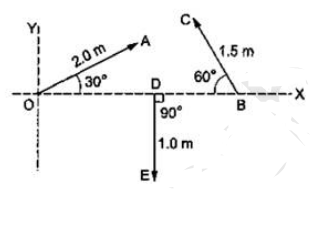Deepak Scored 45->99%ile with Bounce Back Crack Course. You can do it too!

# Refer to the figure. (a) Find the magnitude,

Question:

Refer to the figure. (a) Find the magnitude, (b) $x$ and $y$ components and (c) the angle with the $\mathrm{X}$-axis of the resultant of $\overrightarrow{O A}, \overrightarrow{B C}$ and $\overrightarrow{D E}$.Solution:

$x$ component of $\mathrm{OA}=2 \cos 30^{\circ}=\sqrt{3}$

$x$ component of $B C=1.5 \cos 120^{\circ}=-0.75$

$x$ component of $D E=1 \cos 270^{\circ}=0$

$y$ component of $\mathrm{OA}=2 \sin 30^{\circ}=1$

component of $B C=1.5 \sin 120^{\circ}=1.3$

component of $\mathrm{DE}=1 \sin 270^{\circ}=-1$

$\mathrm{Rx}=\mathrm{x}$ component of resultant $=3-0.75+0=0.98 \mathrm{~m}$

$\mathrm{Rx}=\mathrm{x}$ component of resultant $=3-0.75+0=0.98 \mathrm{~m}$

Ry $=$ resultant $y$ component $=1+1.3-1=1.3 \mathrm{~m}$

So, $R=\left(R_{x}{ }^{2}+R_{y}{ }^{2}\right)^{1 / 2}=1.6 \mathrm{~m}$

It makes and angle $\theta$ with positive $x$-axis then $\tan \theta=R_{y} / R_{x}=1.32 \theta=\tan ^{-1}(1.32)$